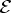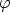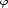DIPS 7/2002 [tex source, PostScript, PDF file, dvi]

Title: On symmetries and cohomological invariants of equations possessing flat representations.

We study the equationfc of flat connections in a given fiber bundle and discover a specific geometric structure on this equation, which we call a flat representation. We generalize this notion to arbitrary PDE and prove that flat representations of an equationare in 1-1 correspondence with morphisms:fc,andfc being treated as submanifolds of infinite jet spaces. We show that flat representations include several known types of zero-curvature formulations of PDEs. In particular, the Lax pairs of the self-dual Yang-Mills equations and their reductions are of this type. With each flat representationwe associate a complex Cof vector-valued differential forms such that H1(C) describes infinitesimal deformations of the flat structure, which are responsible, in particular, for parameters in Baecklund transformations. In addition, each higher infinitesimal symmetry S ofdefines a 1-cocycle cS of C. Symmetries with exact cS form a subalgebra reflecting some geometric properties ofand. We show that the complex corresponding tofc itself is 0-acyclic and 1- acyclic (independently of the bundle topology), which means that higher symmetries offc are exhausted by generalized gauge ones, and compute the bracket on 0-cochains induced by commutation of symmetries.

24 pages, LaTeX-2e (AmSLaTeX) and diagrams.tex.

To compile source files (.tex) one needs the title page style files titlatex.tex.